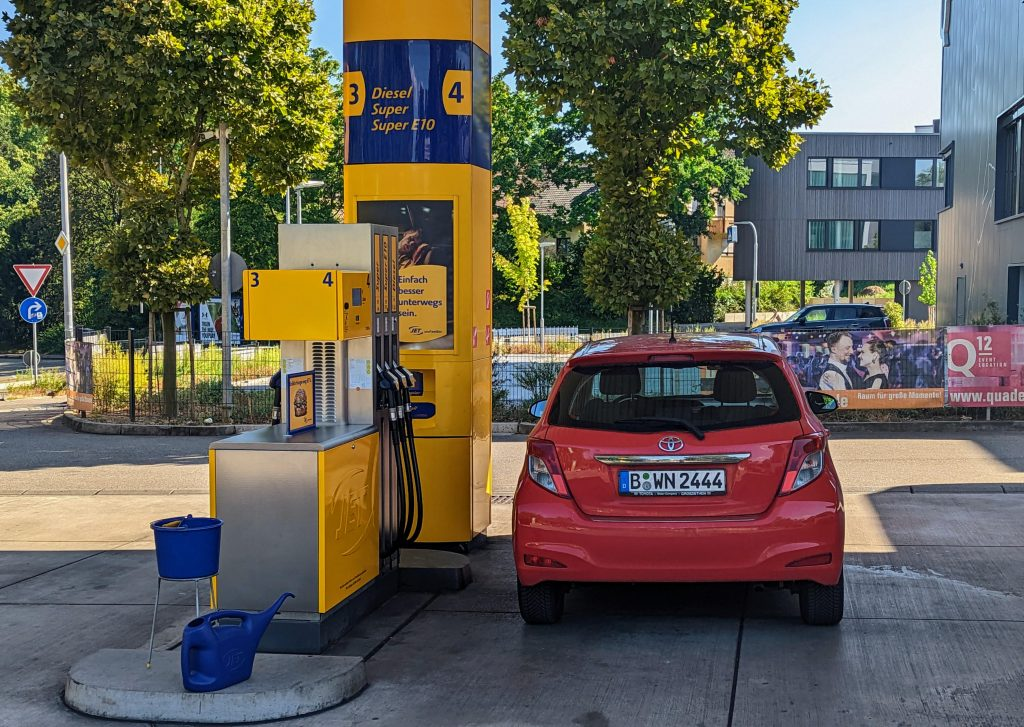# Gas Station Power Output

· Timo Denk

A gas station does not produce energy, but it transfers it into cars. The energy is in the form of gasoline. As we will see in this article, one might intuitively underestimate just how much energy can be stored in gasoline.

Uncle Thorsten raised an interesting question: If a gas station fills up the tanks of several cars simultaneously, how much energy is it transferring? Or in other words: What’s the gas station’s power output? Clearly, the gas station does not produce the energy, but appreciating how it can output a lot of energy so quickly is fascinating. So let’s dig into the numbers…A random gas station in Waiblingen needs about 40s to fill the tank of my dad’s car. It took in 25 liters.

The volumetric flow rate is

$$\dot V=\frac{25\text{l}}{40\text{s}}\approx 0.6\frac{\text{l}}{\text{s}},.$$

The combustion of a liter of gasoline releases about $34.2\text{MJ}$ of energy [see this table on Wikipedia]. Multiplying volumetric flow rate and energy density yields the amount of energy flowing from gas station to car:

$$P=0.6\frac{\text{l}}{\text{s}}\times34.2\frac{\text{MJ}}{\text{l}}=20.52\frac{\text{MJ}}{\text{s}}=20.52\text{MW},.$$

In comparison, a Tesla supercharger outputs a peak of $150\text{kW}$. A mere 0.7% of the gasoline car’s energy intake. Needless to say that electric cars are still cooler than combustion engine cars, but in terms of charging speed they lack behind.Back to the gas station: How much energy does it output in total? A small one – like the one we visited today to measure the flow rate (and refuel the car) – has six fuel dispensers. It would transfer a whopping $123\text{MJ}$ of energy per second. That is, its power is $123\text{MW}$.The Hoover dam in comparison has an installed capacity of $2080\text{MW}$ [Wikipedia]. In practice the dam does not operate at that capacity. In fact its water levels are at an all-time low right now. Averaged over a year, the dam’s power station has around $376\text{MW}$. That’s the same order of magnitude as a gas station!

As a last data point for comparison, consider nuclear power plants. An average one in the US outputs about $1\text{GW}$ [energy.gov]. As few as eight (!) of the inconspicuous gas stations can already transfer all the energy into car tanks, which a nuclear power plant outputs at a given time! That’s just mind-blowing. The calculations illustrate just how much energy fossil fuel can store and what a crazy process the common activity of refueling a car actually is.